# Class 12 Maths NCERT Solutions for Chapter 5 Continuity and Differentiability Exercise 5.6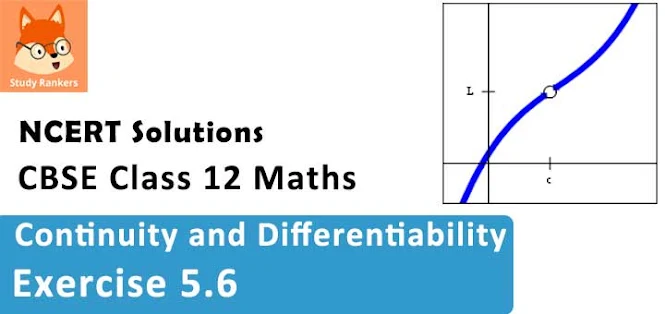### Continuity and Differentiability Exercise 5.6 Solutions

1. If x and y are connected parametrically by the equation, without eliminating the parameter, find dy/dx .
x = 2at2 , y = at4

Solution

The given equations are x = 2at2 , y = at4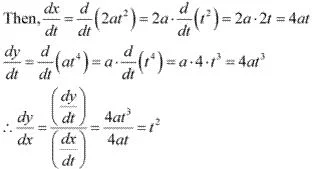2. If x and y are connected parametrically by the equations x = a cos θ, y = b cos θ, without eliminating the parameter, find dy/dx .

Solution

The given equations are x = a cos θ, y = b cos θ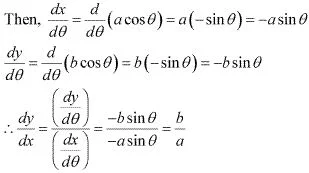3. If x and y are connected parametrically by the equation, without eliminating the parameter, find dy/dx .
x = sin t, y = cos 2t

Solution

The given equations are x = sin t, y = cos 2t

Solution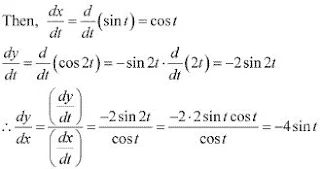4. If x and y are connected parametrically by the equation, without eliminating the parameter, find dy/dx.
x = 4t, y = 4/y
Solution
The given equations are x = 4t, y = 4/y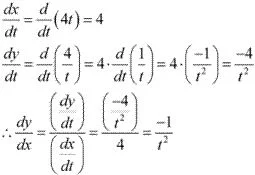5. If x and y are connected parametrically by the equation, without eliminating the parameter, find dy/dx.
x = cos θ- cos 2θ, y = sin θ - sin 2θ
Solution
The given equations are x = cos θ- cos 2θ, y = sin θ - sin 2θ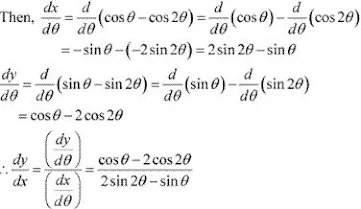6. If x and y are connected parametrically by the equation, without eliminating the parameter, find dy/dx.
x = a (θ – sin θ), y = a (1 + cos θ)

Solution

The given equations are x =  a (θ – sin θ), y = a (1 + cos θ)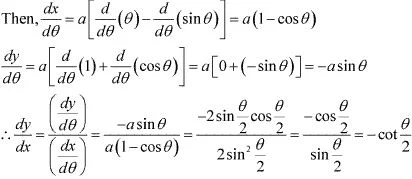7. If x and y are connected parametrically by the equation, without eliminating the parameter, find dy/dx.
x = sin3t/√(cos 2t) , y = cos3t/√(cos 2t)

Solution

The given equations are x = sin3t/√(cos 2t) and  y = cos3t/√(cos 2t)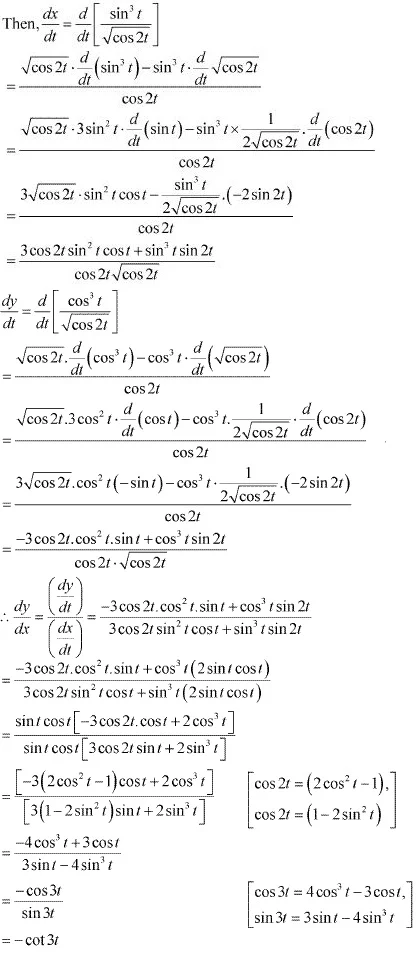8. If x and y are connected parametrically by the equation, without eliminating the parameter, find dy/dx.
x = a[cos t + log tan (t/2) ], y = a sin t

Solution

The given equation are x = a[cos t + log tan (t/2) ], y = a sin t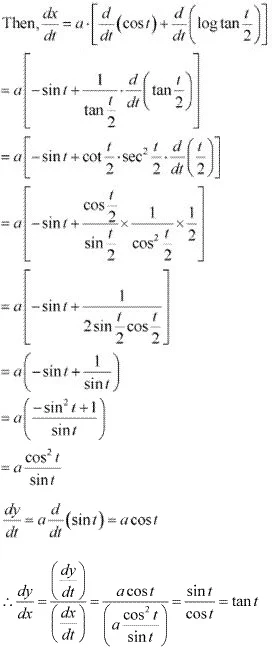9. If x and y are connected parametrically by the equation, without eliminating the parameter, find dy/dx.
x = a sec θ, y = b tan θ

Solution

The given equations are x = a sec θ, y = b tan θ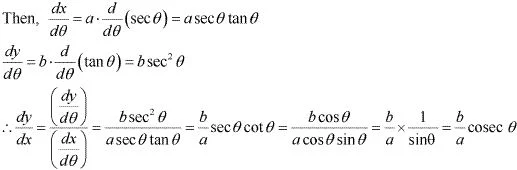10. If x and y are connected parametrically by the equation, without eliminating the parameter, find dy/dx.
x = a (cos θ + θ sin θ), y = a (sin θ – θ cos θ)

Solution

The given equations are x = a (cos θ + θ sin θ), y = a (sin θ – θ cos θ)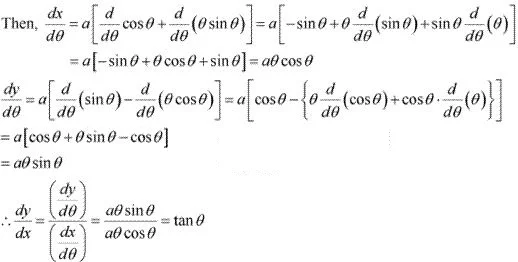11. If x = √(asin – 1), y = √(acos – 1) show that dy/dx = -y/x

Solution

The given equations are  x = √(asin – 1) and  y = √(acos – 1)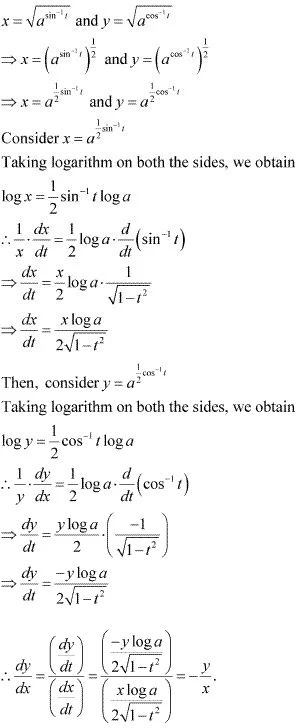Hence, proved.